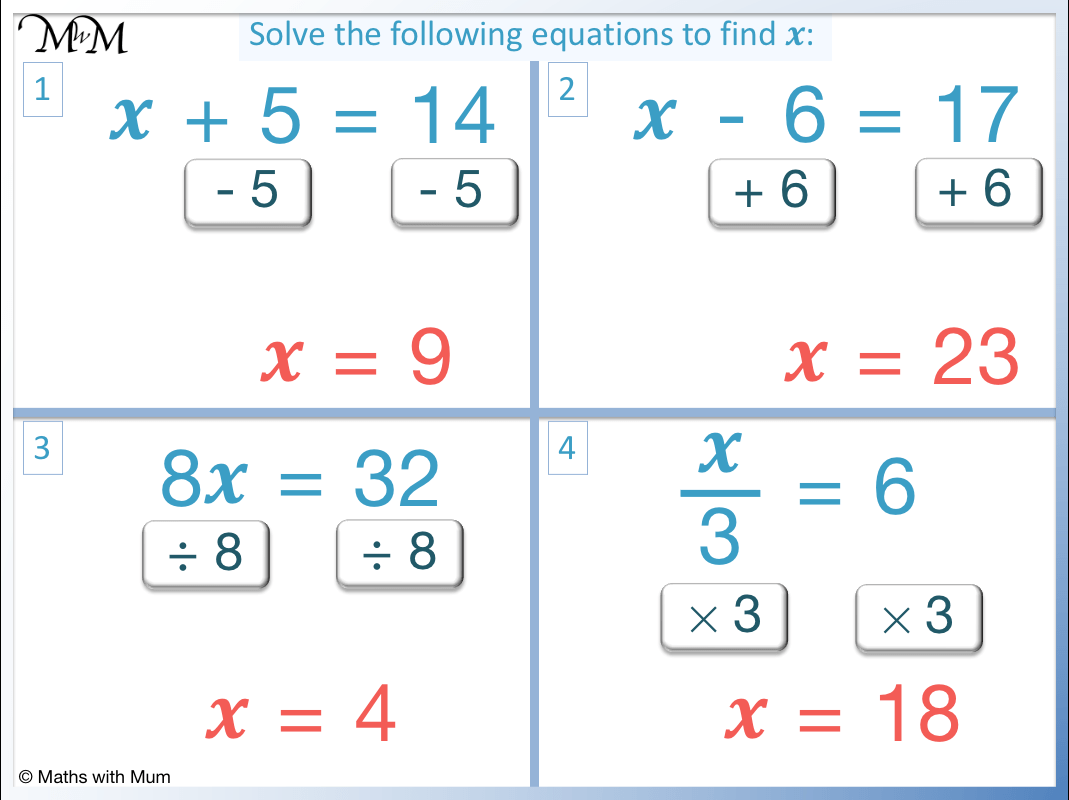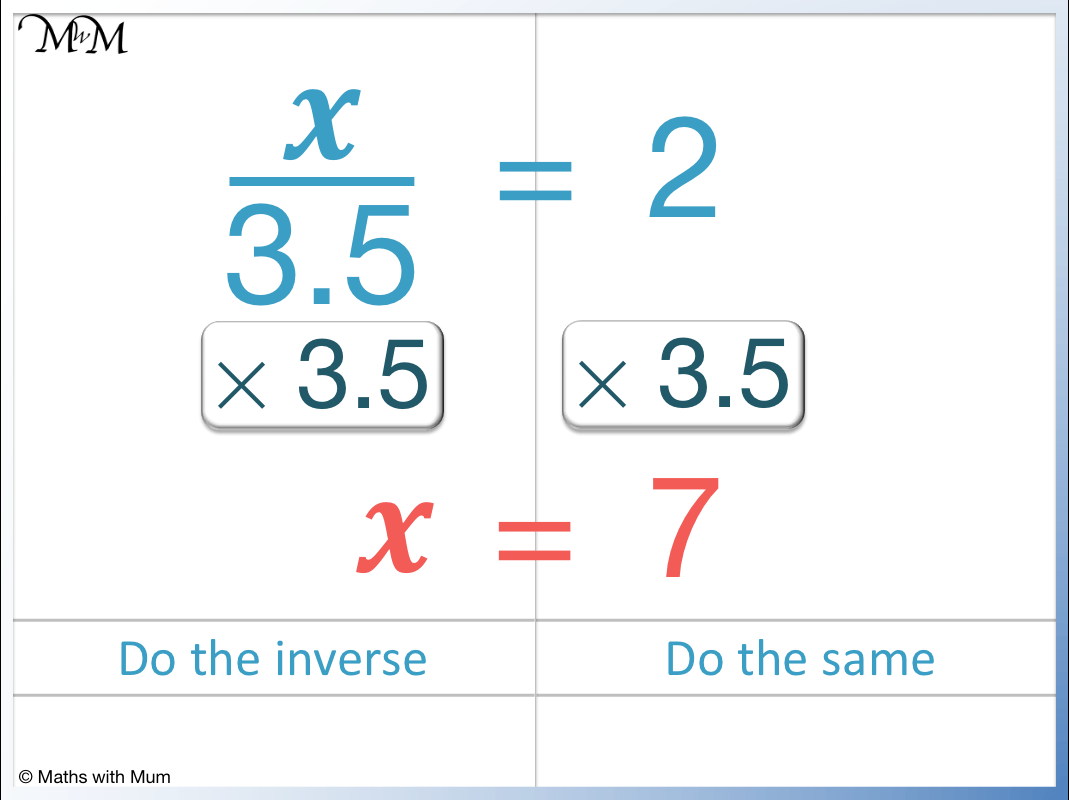# How to Solve One-Step Equations

How to Solve One Step Equations• The 4 different types of one step equation are shown above.
• To solve one step equations, we want the variable (𝑥) on its own on one side of the equals sign.
• To solve the equations, we use the inverse (opposite) of the operation that is acting on the variable.
• To solve an equation that contains an addition, we use subtraction.
• To solve 𝑥 + 5 = 14, subtract the 5 from both sides to leave 𝑥 = 9.
• To solve an equation that contains a subtraction, we use addition.
• To solve 𝑥 – 6 = 17, add the 6 to both sides to leave 𝑥 = 23.
• To solve an equation that contains a multiplication, do a division.
• To solve 8𝑥 = 32, divide both sides by 8 to leave 𝑥 = 4.
• To solve an equation that contains a division (as a fraction), use multiplication.
• To solve   𝑥/3 = 6, multiply both sides by 3 to leave 𝑥 = 18.
To solve a one-step equation, do the opposite operation to what is acting on the variable.

You must do the same thing to both sides of the equation.• Here is the one-step equation   𝑥/3 = 4.
•   𝑥/3 = 4 means 𝑥 ÷ 3 = 3.
• We want to remove the ÷ 3 on the left of the equals sign so that 𝑥 is the only thing remaining.
• To solve this equation, we use the inverse of division, which is multiplication.
• We multiply both sides of the equation by 3.
• Multiplying the left hand side of the equation by 3 cancels out the division by 3 that is shown by the fraciton. Just 𝑥 remains.
• Because we multiplied the left hand side of the equals sign by 3, we also must multiply the right hand side of the equals sign by 3 to keep the equation balanced.
• 4 × 3 = 12 and so, 𝑥 = 12.# One Step Equations

## What is a One-Step Equation?

A one-step equation is an equation that only involves one mathematical operation to solve. A single addition, subtraction, multiplication or division is used to find the value of the variable. Because only one operation is needed, one-step equations are used to introduce the concept of solving equations.

The four types of one-step equation are:

2. Subtraction
3. Multiplication
4. Division
5. These solving the 4 different types of one step equations are shown in the image below.We can see that the variable 𝑥 plus a number can be solved by subtracting this number.

We can see that the variable x subtract a number can be solved by adding this number.

When the variable has a number in front of it, it is being multiplied by that number. We must divide by this number to solve the equation.

When the variable is written in a fraction with a number below it as the denominator, we must multiply by this denominator to solve the equation.

For example, 𝑥 – 8 = 3 is an exampe of a one-step equation. It can be solved in one-step by adding 8 to both sides. 3 + 8 = 11 and so, 𝑥 = 11.## How to Solve One-Step Equations

To solve one-step equations, use the inverse (opposite) of the operation that is acting on the variable. For example, if there is an addition, use subtraction, if there is a subtraction use addition, if there is multiplication, use division and if there is division, use multiplication. This is done to both sides of the equals sign in order to leave the variable on its own on one side of the equals sign.

For example, 𝑥 + 2 = 6 is a one-step equation involving an addition. We will solve it with a subtraction.The opposite of adding 2 is to subtract 2 to both sides of the equation.

𝑥 + 2 – 2 = 𝑥.

Subtracting 2 has the effect of removing the + 2.

Because we subtracted 2 to the left hand side of the equation, we subtract 2 from the right hand side of the equation. 6 – 2 = 4.

Therefore 𝑥 = 4.

Here is another example of solving a one-step equation.

We have 2𝑥 = 10. Here, 2𝑥 means 2 × 𝑥. The variable 𝑥 is multiplied by 2.

We want to remove the multiplication by 2 to leave just 𝑥. We will do the opposite of multiplying by 2, which is to divide by 2.2𝑥 ÷ 2 = 𝑥 and 10 ÷ 2 = 5.

Therefore 𝑥 = 5.

## How to Solve One-Step Equations with Addition

To solve a one-step equation that contains an addition of a number, subtract this number from both sides of the equation. For example, in 𝑥 + 7 = 15, we remove the + 7 by subtracting 7 from both sides to leave 𝑥 = 8.On the left hand side, 𝑥 + 7 – 7 = 𝑥.

On the right hand side, 15 – 7 = 8.

We have subtracted 7 from both sides to get 𝑥 = 8.

## How to Solve One-Step Equations with Subtraction

To solve a one-step equation that contains the subtraction of a number, add this number from both sides of the equation. For example, in 𝑥 – 2 = 3, add 2 to both sides to get 𝑥 = 5.On the left hand side, 𝑥 – 2 + 2 = 𝑥. Adding 2 removes the -2.

On the right hand side, 3 + 2 = 5.

The one-step equation is solved to leave 𝑥 = 5.

## How to Solve One-Step Equations with Multiplication

A one-step equation involving multiplication will have a number written in front of the variable. To solve this equation, divide both sides of the equation by this number. For example, in 2𝑥 = 10, the variable is multiplied by 2. To remove the 2, divide both sides of the equation by 2 to leave 𝑥 = 5.2𝑥 means 2 multiplied by 𝑥. To remove the multiplication by 2, divide by 2 to leave 𝑥.

We must also divide the right hand side of the equation by 2. 10 ÷ 2 = 5.

Therefore 𝑥 = 5.

## How to Solve One-Step Equations with Fractions

A one-step equation with a fraction involves dividing the variable on top of the fraction by the number on the bottom of the fraction. To solve this equation, multiply both sides of the equation by the number on the bottom of the fraction. For example,   𝑥/9 = 5 can be solved by multiplying both side of the equation by 9 to get 𝑥 = 45.In the equation   𝑥/9 = 5, the denominator on the bottom of the fraction is 9. This means that 𝑥 is being divided by 9.

To remove the fraction and leave just 𝑥 on its own, we must multiply by 9. This has the effect of cancelling out the division by 9.

Because we multiplied the left-hand side by 9, we also multiply the right-hand side by 9. 5 multiplied by 9 is 45 and so, 𝑥 = 45.

Here is another example of an equation containing a fraction. We have   𝑥/3 = 4.The denominator of the fraction is 3 and so,   𝑥/3   means 𝑥 ÷ 3.

The inverse of dividing by 3 is to multiply by 3.

On the left hand side of the equation,   𝑥/3   × 3 = 𝑥. This is because dividing by 3 and multiplying by 3 cancel out.

What we do to one side of the equation, we must do to the other and so, we multiply 4 by 3.

4 × 3 = 12 and therefore, 𝑥 = 12.

## One-Step Equations with Decimals

Here are some examples of solving one-step equations with decimals.

Here is 𝑥 + 2.4 = 5.5.

To remove the decimal of 2.4, we subtract 2.4 from both sides.5.5 – 2.4 = 3.1 and so, 𝑥 = 3.1

Here is 𝑥 – 3.6 = 1.2. We have decimals on both sides of this one-step equation.To remove the subtraction of the decimal – 3.6, we must add 3.6 to both sides.

𝑥 = 4.8.

In the example of 1.5𝑥 = 6, the variable is multiplied by a decimal. We must divide by this decimal to remove it.Dividing 1.5𝑥 by 1.5 just leaves 𝑥.

To divide 6 by 1.5, we can think how many times 1.5 goes into 6. 1.5 goes into 6 four times and so 𝑥 = 4.

Here we have   𝑥/3.5 = 2. We have a decimal on the denominator of this one-step equation.We simply need to multiply both sides of the equation by 3.5.

2 × 3.5 = 7 and so, 𝑥 = 7.Now try our lesson on How to Find the Mean where we learn how to find the mean of a set of numbers.error: Content is protected !!